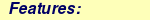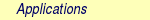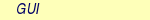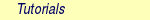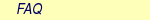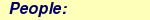MOLCAS manual:Next: 6.23 GRID_IT: A Program Up: 6. Program Based Tutorials Previous: 6.21 VIBROT

Subsections

# 6.22 SINGLE_ANISO -- A Magnetism of Complexes Program

The program SINGLE_ANISO calculates nonperturbatively the temperature- and field- dependent magnetic properties (Van Vleck susceptibility tensor and function, molar magnetization vector and function) and the pseudospin Hamiltonians for Zeeman interaction (the g tensor and higher rank tensorial components) and the zero-field splitting (the D tensor and higher rank tensorial components) for arbitrary mononuclear complexes and fragments on the basis of ab initio spin-orbit calculations. SINGLE_ANISO requires as input file the RUNFILE containing all necessary ab initio information: spin orbit eigenstates, angular momentum matrix elements, the states been mixed by the spin-orbit coupling in RASSI, etc. Usually, the SINGLE_ANISO runs after RASSI.

For a proper spin-orbit calculation the relativistic basis sets should be used for the whole calcualtion. For SEWARD, the atomic mean-field (AMFI), Douglas-Kroll (DOUG) must be employed. To ensure the computation of angular momentum integrals the ANGMOM should be also used, specifying the origin of angular momentum integrals as the coordinates of the magnetic center of the molecule, i.e. the coordinates of the atom where the unpaired electrons mainly reside. For program RASSI the necessary keywords are: SPIN, since we need a spin-orbit coupling calculation, and MEES, to ensure the computation of angular momentum matrix elements in the basis of spin-free states (SFS).

In the cases where spin-orbit coupling has a minor effect on the low-lying energy spectrum (most of the isotropic cases: Cr3+, Gd3+, etc.) the pseudospin is usually the same as the ground spin. For these cases the SINGLE_ANISO may run without specifying any keywords in the input file.

&SINGLE_ANISO

In the cases when spin-orbit coupling play an important role in the low-lying energy spectrum, i.e. in the cases of e.g. octahedral Co2+, most of the lanthanide complexes, the pseudospin differs strongly from the spin of the ground state. In these cases, the dimension of the pseudospin can be found by analysing the spin-orbit energy spectrum obtained at RASSI. The pseudospin is best defined as a group of spin-orbit states close in energy. Once specified, these eigenstates are further used by the SINGLE_ANISO to build proper pseudospin eigenfunctions. As an example of an input for SINGLE_ANISO requiring the computation of all magnetic properties (which is the default) and the computation of the g tensor for the ground Kramers doublet (i.e. pseudospin of a Kramers doublet is S=1/2).

&SINGLE_ANISO
MLTP
1
2

SINGLE_ANISO has implemented pseudospins: S=1/2, S=1, ..., up to S=7/2. The user can also ask for more pseudospins at the same time:

&SINGLE_ANISO
MLTP
3
2  4  2
For the above input example, the SINGLE_ANISO will compute the g tensor for the ground Kramers doublet (spin-orbit states 1 and 2), the g tensor, ZFS tensor and coefficients of higher rank ITO for the pseudospin S=3/2 (spin orbit functions 3-6), and the g tensor for the third excited Kramers doublet (spin orbit functions 7 and 8).

## 6.22.1 SINGLE_ANISO Output

The SINGLE_ANISO section of the MOLCAS output is divided in four parts. In the first part, the g tensor and higher rank Zeeman tensors are computed. They are followed by D tensor and higher rank ZFS tensors. The program also computes the angular moments in the direction of the main magnetic axes.

In the second part, the paramaters of the crystal field acting on the ground atomic multiplet of lanthanides are calculated.

In the third part, the powder magnetic susceptibility is printed, followed by the magnetic susceptibility tensors with and without intermolecular interaction included.

In the fourth part, magnetization vectors (if required) are printed, and then the powder molar magnetization calculated for the TMAG temperature.

The keywords TINT and HINT control the temperature and field intervals for computation of magnetic susceptibility and molar magnetization respectively. Computation of the magnetic properties at the experimental temperature and field points with the estimation of the standard deviation from experiment is also possible via TEXP, defining the experimental temperature and measured magnetic susceptibility and HEXP, defining the experimental field and averaged molar magnetization.

&SINGLE_ANISO
TITLE
g  tensor  and  magnetic  susceptibility
TYPE
4
MLTP
2
3  3
TINT
0.0  100  101  0.001

The above input requires computation of the parameters of two pseudospins S=1: the ground (spin-orbit functions 1-3) and first excited (spin-orbit functions 4-6) and the magnetic susceptibility in 101 steps equally distributed in the temperature domain 0.0-100.0 K.

## 6.22.2 SINGLE_ANISO - Basic and Most Common Keywords

 Keyword Meaning MLTP Specifies the number and dimension of the pseudospins Hamiltonians TMAG Sets the temperature for the computation of molar magnetization MVEC Number and radial coordinates of directions for which the magnetization vector will be computed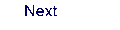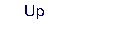Next: 6.23 GRID_IT: A Program Up: 6. Program Based Tutorials Previous: 6.21 VIBROT